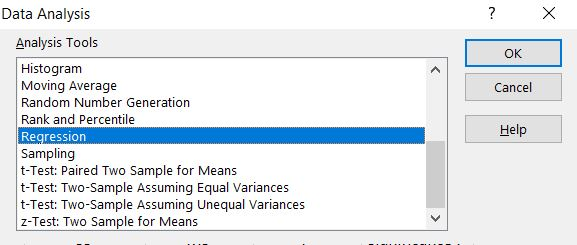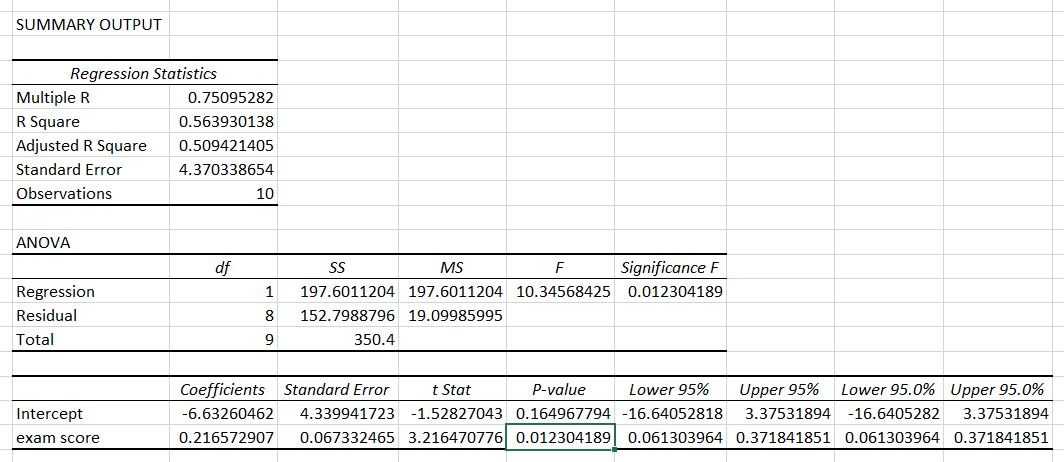### How To Calculate P Value In Excel

In this Excel tutorial you will teach yourself how to calculate p value in Excel.

P-value is a probability that the given result is due to chance.

The correct interpretation of the statistical significance score P tells about the probability of getting the difference we see in our study, or even greater if the null hypothesis is actually true.

## How to calculate p value in Excel?

To calculate p-value use Data Analysis Toolpak add-in.

`Here's how to add the analysis toolpak on excel.`

First prepare your data. I have two sets of value how many hours students studied to the exam and their exam scores.

Click Data Analysis button and choose Regression.Put input values. In my example Y Range are hours studied and X Range is for exam scores.

As and Output Range choose where would you like to put results of Regression analysis in your sheet.Here there are results of regression analysis.P value of exam score is exactly 0.012304189.

`You got also other values like Standard Error and Anova test.`

## How to interpret p value?

See the p value:

• Significant: <=0.05
• Marginally significant: <=0.1
• Insignificant: >0.1

In my example it is significant because 0.012304189 is less than 0.05. It was worth to study hard to get better exam score.

## Template

```Further reading: